### Home > A2C > Chapter 10 > Lesson 10.2.2 > Problem10-79

10-79.
1. What do you think 0! is equal to? Homework Help ✎

1. Try it on your calculator to see what you get.

2. What does 8P8 mean? What should 8P8 be equal to? Write 8P8 using the factorial formula. Why is it necessary for 0! to equal 1?

3. Do you remember how to show that 20 = 1? You can use a sequence of powers of 2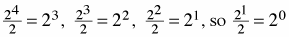, but we also know that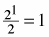. Therefore 20 = 1.
You can construct a similar pattern for 0!, starting with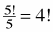and then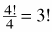Continue the pattern and make an argument to justify that 0! = 1.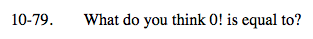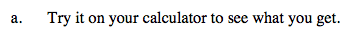You should get 1.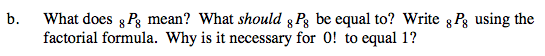Refer to the Math Notes box from Lesson 10.2.3 for help.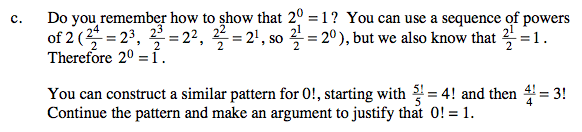$\frac{3!}{3}=2!,\,\frac{2!}{2}=1!,\,\frac{1!}{1}=0!$